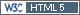Elena777
Junior

Belarus,
2018-01-31 13:53

Posting: # 18315
Views: 1,484

## expsampleN.TOST vs. sampleN.TOST [R for BE/BA]

Hello to everyone! I would like to get information about difference between functions expsampleN.TOST and sampleN.TOST. I've read topics at this forum which were contained some data about function expsampleN.TOST. As I noticed expsampleN.TOST is used when a pilot study was conducted and we are able to input the data from that study (estimated CV, m or df, design) to launch the function and to get a result.

So I have 4 questions:
1. The fundamental difference between expsampleN.TOST and sampleN.TOST?
2. CV in function expsampleN.TOST is CVintra, isn't it?
3. In both cases we can choose the value of desired power (for example 0,8 or 0,9). But in description of function expsampleN.TOST the power is characterized as "expected" (sample size based on expected power) and in description of function sampleN.TOST the power is characterized as "given" (calculates the necessary sample size to have at least a given power). What is the difference between expected and given power?
4. Can I use expsampleN.TOST when I did not conduct a pilot study but I have data from other BE studies, information about which was published? If yes, function expsampleN.TOST seems to have advantages over function sampleN.TOST because in a former case I can take into account uncertainty of CV (as we know CVintra is characterized as a parameter with some degree of uncertainty in general sence).

Ben
Regular

2018-02-01 18:48

@ Elena777
Posting: # 18325
Views: 1,151

## expsampleN.TOST vs. sampleN.TOST

Dear Elena777,

Let me try to answer your questions.

» 1. The fundamental difference between expsampleN.TOST and sampleN.TOST?

`expsampleN.TOST` uses the "expected power" as target power, whereas `sampleN.TOST` uses the classical power as target power to determine the sample size. That is, `expsampleN.TOST` allows you to take uncertainty of either the CV or the gMean ratio or even both into account when determining the sample size of your trial. Usually this will give you a higher required sample size as compared to `sampleN.TOST`. Note that my last sentence assumes that the value for "targetpower" is the same for both `sampleN.TOST` and expsampleN.TOST. This may not be fair because for `expsampleN.TOST` it may be reasonable to choose a lower level.

» 2. CV in function expsampleN.TOST is CVintra, isn't it?

Depends on the design argument. If `design="parallel"` then it is the total CV, otherwise it is the intra CV as you said.

» 3. In both cases we can choose the value of desired power (for example 0.8 or 0.9). But in description of function expsampleN.TOST the power is characterized as "expected" (sample size based on expected power) and in description of function sampleN.TOST the power is characterized as "given" (calculates the necessary sample size to have at least a given power). What is the difference between expected and given power?

I think here is a misunderstanding. The important thing in "given power" is not the word "given", but the word "power": the description for `expsampleN.TOST` could also be "... to have at least a given expected power". The sample size functions simply iterate until you have a sample size with which you have either a classical power (`sampleN.TOST`) or expected power (`expsampleN.TOST`) greater or equal to the specified value in the argument "targetpower". For the definitions of "power" and "expected power" refer for example to the documentation within the PowerTOST source (inst/doc/ BE_power_sample_size_excerpt.pdf and Expected_Power_for_TOST.pdf, respectively).

» 4. Can I use expsampleN.TOST when I did not conduct a pilot study but I have data from other BE studies, information about which was published? If yes, function expsampleN.TOST seems to have advantages over function sampleN.TOST because in a former case I can take into account uncertainty of CV (as we know CVintra is characterized as a parameter with some degree of uncertainty in general sence).

`exppower.TOST` and `expsampleN.TOST` have been developed based on the idea that you have performed a pilot trial. However, you can also use it in such a way that you do not directly assume you have one prior pilot trial. So the answer is 'yes' (with some limitation, see my example below). What matters is the specification of the prior distribution for the calculation of the expected power. The functions for expected power and sample size also allow you to directly specify "`df`" and/or "`SEM`" (via the `prior.parm` argument) - those do not rely on a specific size m (or design) of a previous trial. Let us imagine the following situation: let's say you have quite some information from the literature about your variability and you have calculated a pooled CV (e.g. using `PowerTOST::CVpooled`) which you assume to be fixed. You only want to take uncertainty wrt the GMR into account and you want to define the prior distribution of your treatment difference. In `exppower.TOST` the prior is assumed to be Normal, and you can specify the standard deviation of this distribution via the `SEM` argument (`prior.parm = list(SEM = xx)`). The mean of this prior will be set to `log(theta0)` where `theta0` is the main argument from `exppower.TOST` (this is a limitation, in a more general setting you could use a different mean for the prior than for planning the new trial, but this is not possible here). Similarly, you can imagine situations for `prior.type = "CV"` or` = "both"`.

I hope this clarifies a bit.

Best regards,
Ben
Bioequivalence and Bioavailability Forum |  Admin contact
19,271 posts in 4,099 threads, 1,315 registered users;
online 11 (0 registered, 11 guests [including 7 identified bots]).

It’s easy to lie with statistics;
it is easier to lie without them.    Frederick Mosteller

The BIOEQUIVALENCE / BIOAVAILABILITY FORUM is hosted byIng. Helmut Schütz